## 题型1

• 判断同步时序电路还是异步时序电路，并写出时钟方程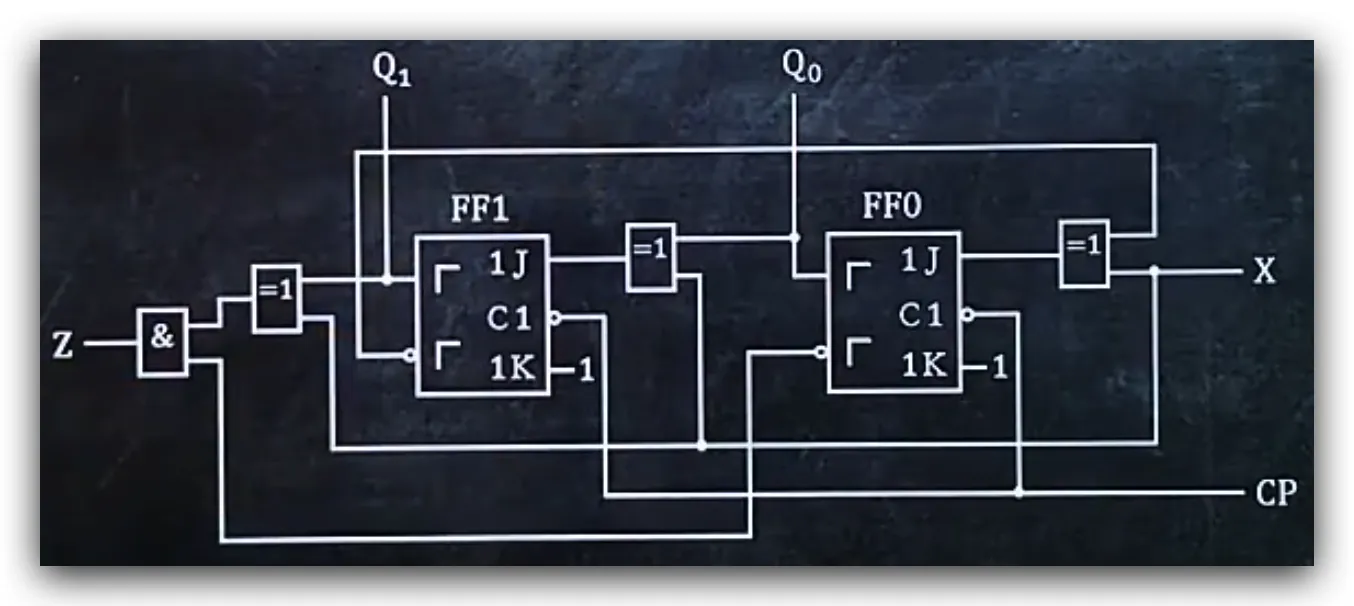1. 判断C1接口是不是连接在同一个电路上
• 如果连接在同一电路上则是同步时序电路
• 如果没有连接在同一电路上则是异步时序电路

1. 时钟方程就是C1接口所连接电路的表达式，格式为`CP=`

CP0=CP
CP1=CP

## 题型2

• 写出时序逻辑电路的输出方程1. 输出方程就是连接Z电路的表达式，格式为`Z=`，结果中如果有Q，需要将Q变为Qn

Z=(Q1n⊕X)·Q0n

## 题型3

• 写出时序逻辑电路的驱动方程1. 驱动方程就是连接J、K、D电路的表达式，格式为，结果中如果有Q，需要将Q变为Qn
• 如果是JK触发器，则只写出`J=``K=`表达式
• 如果是D触发器，则只写出`D=`表达式

J0=X⊕Q1n
K0=1

J1=Q0n⊕X
K1=1

## 题型4

• 写出时序逻辑电路的次态方程1. 写出各个触发器的特性方程
• JK触发器：Qn+1=JQn+KQn
• D触发器：Qn+1=D

Q0n+1=J0Q0n+K0Q0n
Q1n+1=J1Q1n+K1Q1n

1. 驱动方程

J0=X⊕Q1n
K0=1

J1=Q0n⊕X
K1=1

1. 将驱动方程带入特性方程

Q0n+1=(X⊕Q1n)Q0n+0·Q0n
=(X⊕Q1n)Q0n

Q1n+1=(X⊕Q0n)Q1n+0·Q1n
=(X⊕Q0n)Q1n

## 题型5

• 作时序逻辑电路的状态转换表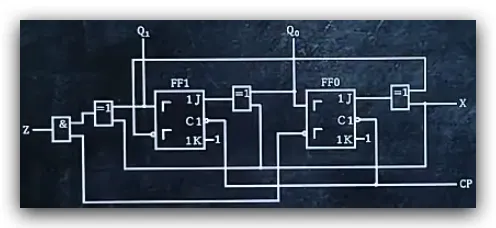1. 次态方程输出方程

Q0n+1=(X⊕Q1n)Q0n+0·Q0n
=(X⊕Q1n)Q0n

Q1n+1=(X⊕Q0n)Q1n+0·Q1n
=(X⊕Q0n)Q1n

Z=(Q1n⊕X)·Q0n

1. 将输出方程和次态方程的异或化简

∵ A⊕B=A·B+A·B
∴ Q0n+1=(X·Q1n+X·Q1n)Q0n
∴ Q1n+1=(X·Q0n+X·Q0n)Q1n
∴ Z=(X·Q1n+X·Q1n)Q0n

1. 分别将X=0和X=1代入式子，化简，然后绘制转换表
• 从Q1n=0，Q0n=0时开始绘制
• 当Q1n+1=0，Q0n+1=0时截止绘制

Q0n+1=(0·Q1n+1·Q1n)Q0n
=Q1nQ0n

Q1n+1=(0·Q0n+1·Q0n)Q1n
=Q0nQ1n

Z=(0·Q1n+1·Q1n)Q0n
=Q1nQ0n

Q1n Q0n Q1n+1 Q0n+1 Z
0 0 0 1 0
0 1 1 0 0
1 0 0 0 1

Q0n+1=(1·Q1n+0·Q1n)Q0n
=Q1nQ0n

Q1n+1=(1·Q0n+0·Q0n)Q1n
=Q0nQ1n

Z=(1·Q1n+0·Q1n)Q0n
=Q1nQ0n

Q1n Q0n Q1n+1 Q0n+1 Z
0 0 1 0 1
1 0 0 1 0
0 1 0 0 0

## 题型6

• 作时序电路的状态图1. 画图

• 画出Q1Q0，并用实线圆圈圈起来，在旁边标注`位置输入量/Z`
• 画出所有现态的Q1nQ0n可能出现的值，并用实线圆圈圈起来
• 当X=0时，将箭头从现态的起始数值循环指向截止数值，在箭头旁标注`0/输出值`
• 当X=1时，将箭头从现态的起始数值循环指向截止数值，在箭头旁标注`1/输出值`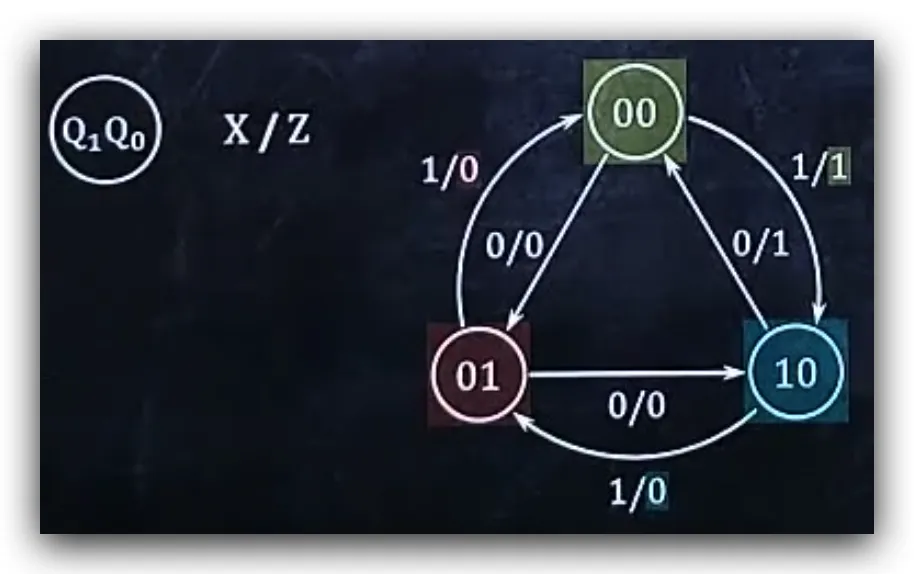## 题型7

• 作时序逻辑电路的时序图1. 根据状态转换表，找出行数，画CP图，突起数为状态转换表行数的2倍1. 原图观察C1接口
• 如果没有圈，则在突起左侧向下画虚线
• 如果有圈，则在突起右侧向下画虚线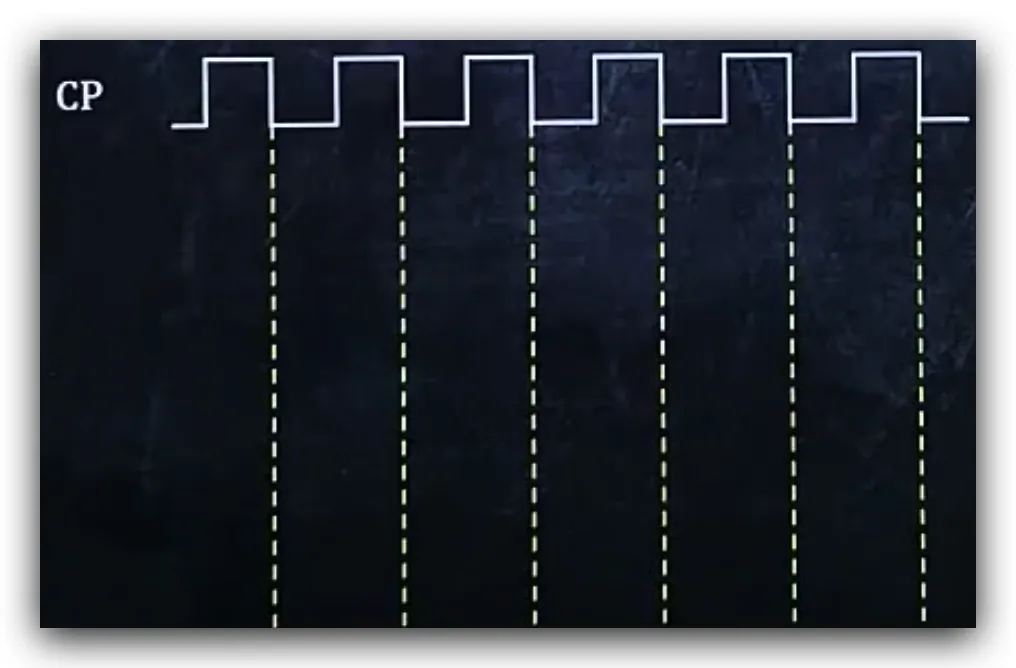1. 在下方画X线，平均分为两段，前半段画0，后半段画1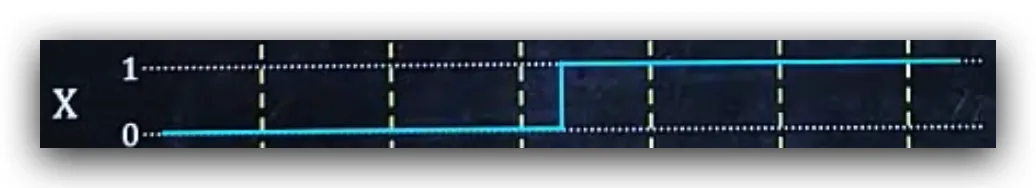1. 在下方画Q1线和Q0线
• 根据当前小段的X值，查看当X等于对应值的状态表
• 根据上一段现态的Q1和Q0的值，得到次态的Q1和Q0的值，将次态的Q1和Q0的值画在Q1线和Q0线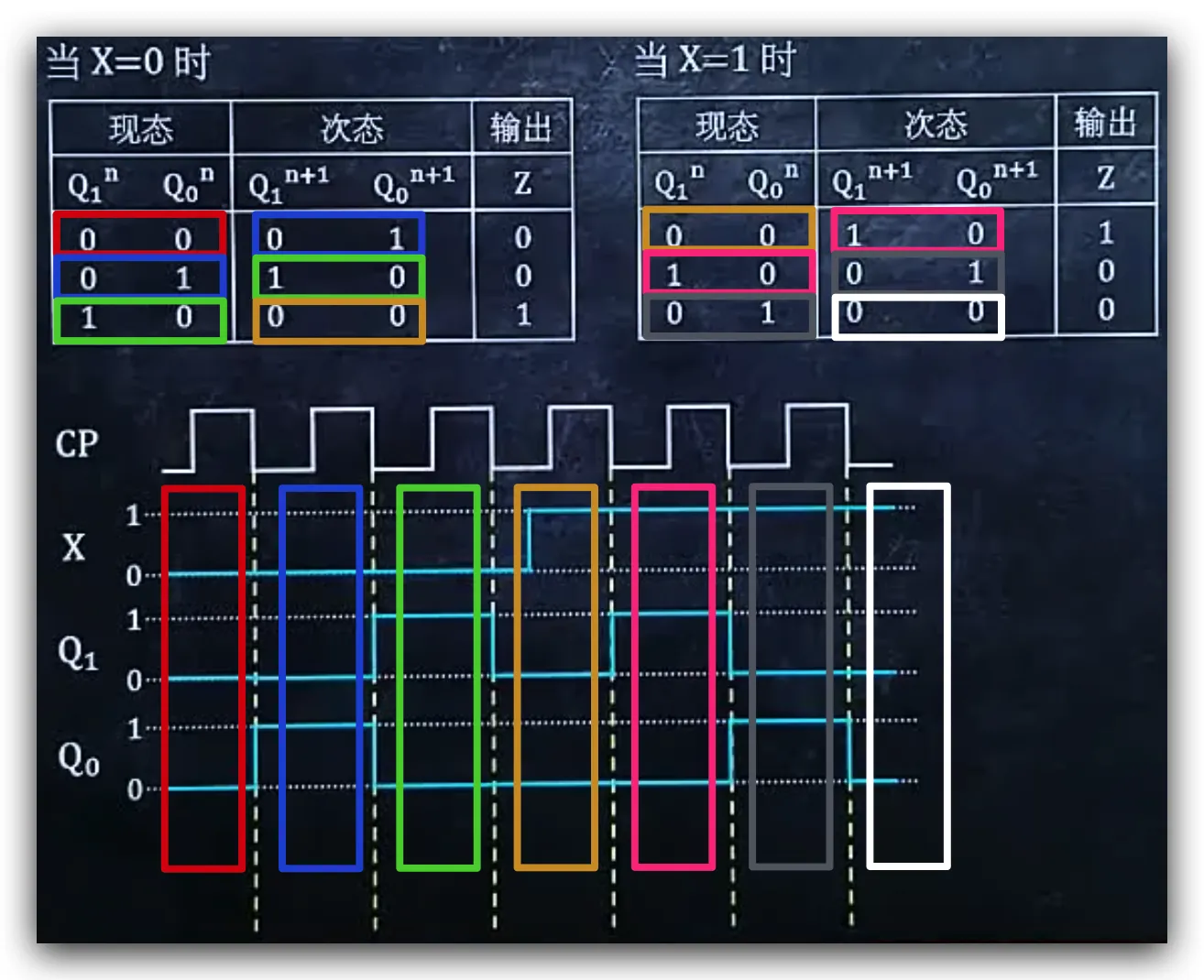1. 将X线中，不对齐的位置，向下画虚线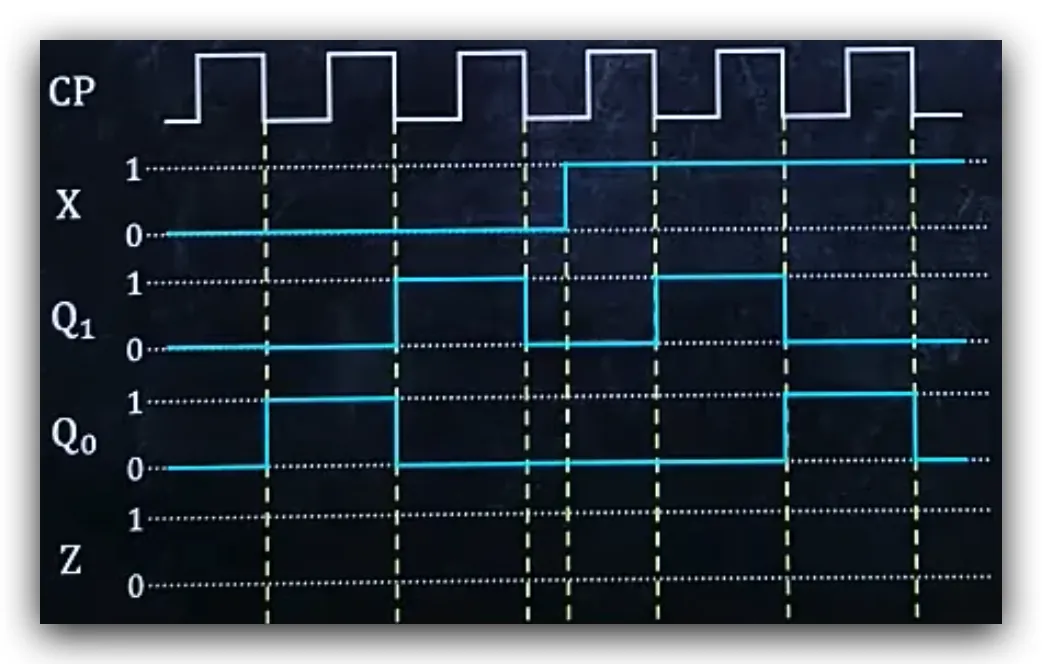1. 在下方画Z线
• 根据当前小段的X值、Q1值、Q0值，得到Z值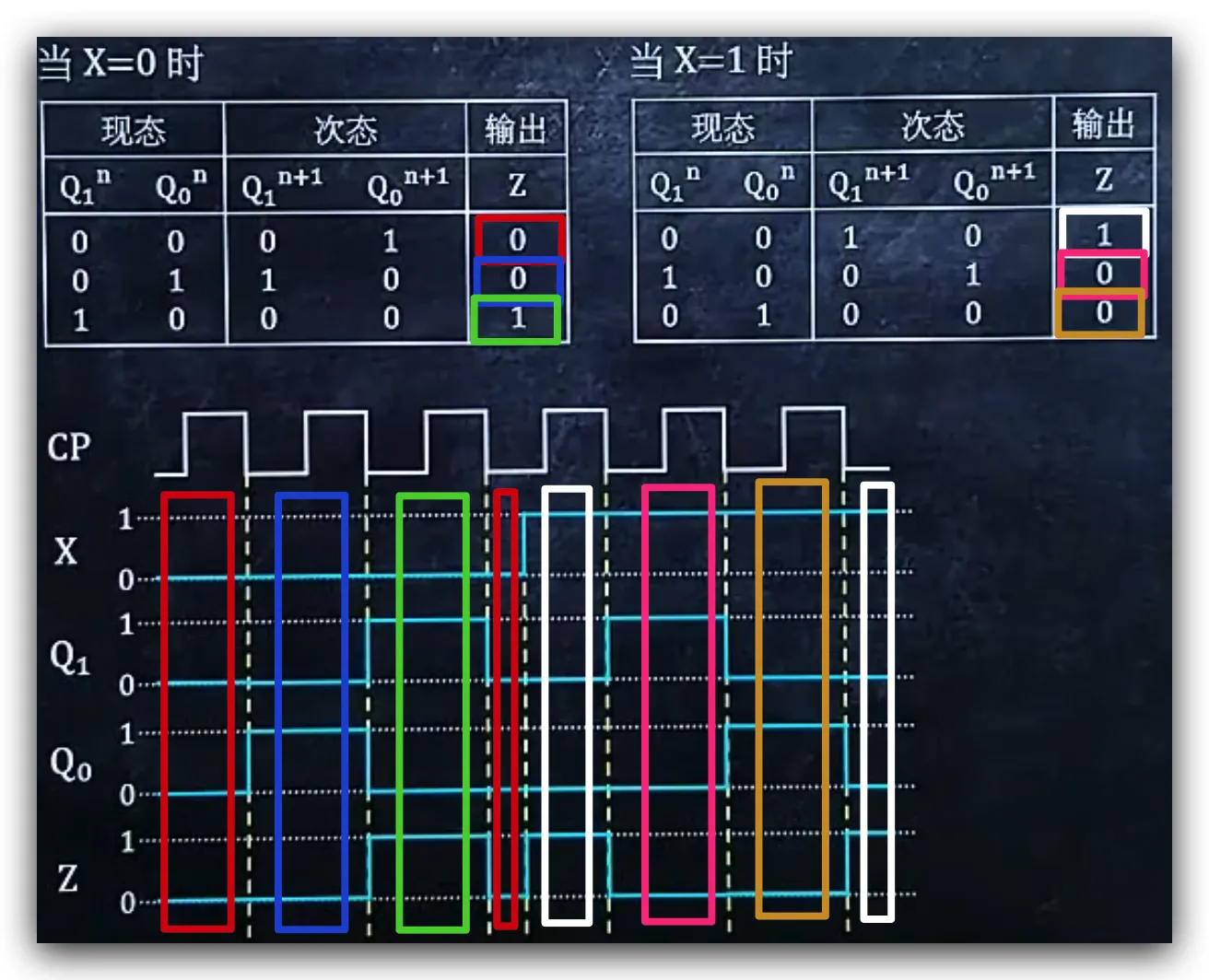## 题型8

• 分析时序逻辑电路的功能1. 状态图

2. 判断状态图中有几个圈被环形箭头指向

• 有几个圈参与环形箭头指向，就是几进制计数器

3进制计数器

1. 判断状态图中的箭头方向是递增还是递减
• 如果递增，就是加计数器
• 如果递减，就是减计数器

## 参考文献微信支付支付宝qqpay比特币moneroethereum